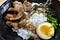# Functional JavaScript — Higher-Order FunctionsPhoto by Héctor J. Rivas on Unsplash

JavaScript is partly a functional language.

To learn JavaScript, we got to learn the functional parts of JavaScript.

In this article, we’ll look at how to use higher-order functions.

# Higher-Order Functions

Higher-order functions are functions that take other functions as arguments and return a function.

It also takes other data and let us manipulate them.

# JavaScript Data Types

JavaScript has several data types.

They include numbers, strings, booleans, objects, `null` and `undefined` .

Functions are data types like strings.

We can pass them around, store them as variables, etc.

This means they act like any other data type.

# Storing a Function

We can store a function in a variable.

For example, we can write:

`let fn = () => {}`

Then we can check its type by using the `typeof` operator:

`typeof fn`

We should get `'function'` returned with that.

# Passing a Function

Functions can have parameters.

So we can write:

`const getType = (arg) => {  console.log(typeof arg)}`

We get the type by using the `typeof` operator from the `arg` .

We can also make our function call a function that’s passed in.

To do that, we can check if we passed in a function to our function.

For example, we can write:

`const getType = (arg) => {  if (typeof arg === "function") {    arg()  } else {    console.log(arg)  }}`

to check if `arg` is a function.

If it’s a function, we call it.

Otherwise, we log its value.

# Returning a Function

We can return a function inside a function.

For instance, we can write:

`let crazy = () => {  return Number}`

We return the built-in `Number` function in our function.

Also, we can call the returned function.

So we can write:

`let fn = crazy();fn("abc");`

We call the function returned by `crazy` .

Then we call `fn` and return the value that it returns.

# Abstraction and Higher-Order Functions

We can abstract the higher-order functions by getting the function from the parameter and calling it.

For example, we can write:

`const forEach = (array, fn) => {  for (const a of array) {    fn(a);  }}`

`fn` is a function, so we can call it.

We loop through the `array` array and call `fn` with each entry of `array` as an argument.

Then we can use it by writing:

`forEach([1, 2, 3], (data) => {  //...})`

We have the array as the first argument.

The 2nd argument is a function that we call on each item.

`forEach` traverses the array and do what we want with it.

Also, we can do the same with an object by writing:

`const forEachObj = (obj, fn) => {  for (const [key, value] of Object.entries(obj)) {    fn(key, value);  }}forEach({  a: 1,  b: 2,  c: 3}, (key, value) => {  //...})`

We created the `forEachObj` function which takes an object and a function as parameters.

Then we loop through the key-value pairs with the `Object.entries` method and call the `fn` function on each `key` and `value` .Photo by Markus Winkler on Unsplash

# Conclusion

We can create higher-order function getting a function and then calling it with a function.

This can be done easily for code that traverses arrays and objects.

Web developer. Subscribe to my email list now at https://thewebdev.info/subscribe/. Email me at hohanga@gmail.com

Web developer. Subscribe to my email list now at https://thewebdev.info/subscribe/. Email me at hohanga@gmail.com